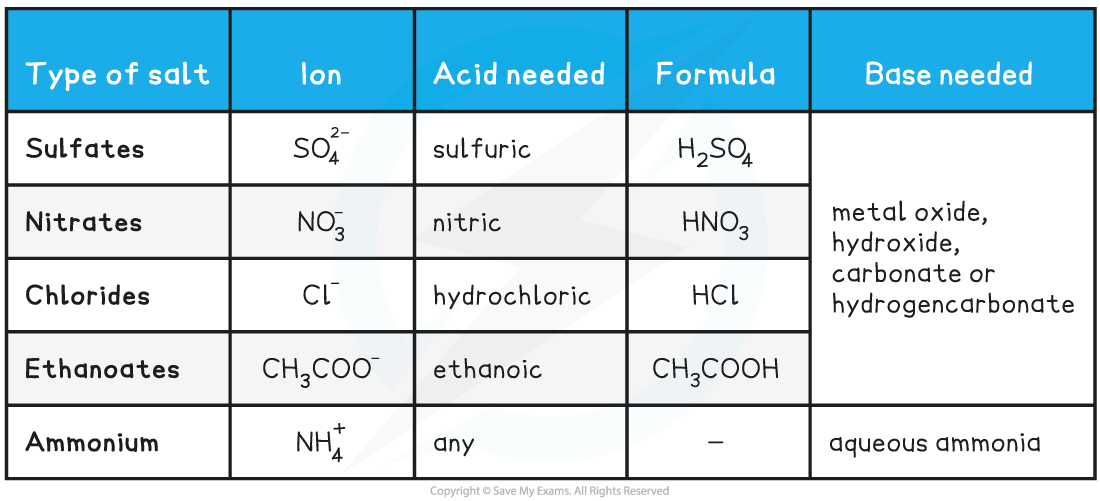# IB DP Chemistry: SL复习笔记8.1.3 Characteristic Reactions of Acids

### Characteristic Reactions of Acids

#### Metals and acids

• The typical reaction of a metal and an acid can be summarized as

acid + metal  →  salt + hydrogen

• For example:

2HCl (aq)       +      Zn (s)  →    ZnCl(aq) +   H2 (g)

hydrochloric acid + zinc    →  zinc chloride + hydrogen

• Clearly, the extent of reaction depends on the reactivity of the metal and the strength of the acid
• Very reactive metals would react dangerously with acids and these reactions are not usually carried out
• Metals low in reactivity do not react at all, for instance copper does not react with dilute acids
• Stronger acids will react more vigorously with metals than weak acids. What signs of reaction would be expected to be different between the two?
• Faster reaction, seen as
• more effervescence
• the metal dissolves faster
• More exothermic

#### Metals and oxides

• The reaction of an acid with a metal oxide forms two products:

acid + metal oxide →  salt + water

• For example:

2HCl (aq)  +      CaO (s)     →        CaCl2 (aq)      +    H2O (l)

hydrochloric acid + calcium oxide →  calcium chloride + water

#### Metals and hydroxides

• The reaction with a metal hydroxide and an acid follows the same pattern as an oxide:

acid + metal hydroxide →  salt + water

• A suitable example might be:

H2SO(aq) +  Mg(OH)2 (s)  →     MgSO(aq)  +    2H2O (l)

sulfuric acid  + magnesium hydroxide →  magnesium sulfate + water

#### Metals and carbonates

• The reaction between a metal carbonate and an acid produces three products:

acid + metal carbonate → salt + water + carbon dioxide

• For example:

2HNO(aq) + CuCO(s)   →    Cu(NO3)2 (aq) + H2O (l) + CO2 (g)

nitric acid + copper carbonate → copper nitrate + water + carbon dioxide

#### Metals and hydrogencarbonates

• The reaction between a metal hydrogencarbonate and an acid is the same as the carbonate reaction with a slight difference in stoichiometry:

acid + metal hydrogencarbonate → salt + water + carbon dioxide

• An example of this would be:

HCl (aq)   +     NaHCO3 (s)     →    NaCl (aq) + H2O (l) + CO2 (g)

hydrochloric acid  + sodium hydrogencarbonate  → sodium chloride + water + carbon dioxide

#### Exam Tip

Make sure you learn the formulae of the common acids and bases and that you can write examples of balanced equations of their characteristic reactions

### Making Salts

• The acids and bases needed to make different salts can be deduced using the principles covered in the previous section
• The table below summarises these reactions

Making Salts Table• Note that although some metals can be used to make salts, they are not classified as bases as water is not a product of the reaction

#### Worked Example

Which are the products of the reaction between zinc oxide and hydrochloric acid?

A. zinc chloride and carbon dioxide

B. zinc chloride, hydrogen gas and water

C. zinc, hydrogen gas and water

D. zinc chloride and water

The correct option is D.

• Metal oxides when reacting with acids produce a salt and water as the only products